Wednesday 17th August 2022
 All India Engineering / Architecture Entrance Examination (AIEEE) CBSE Guess > AIEEE > AIEEE Papers > 2007 > Mathematics AIEEE 2007 Mathematics Q. 1. In a geometric progression consisting of positive terms, each term equals the sum of the next two terms. Then the common ratio of this progression equals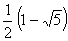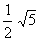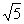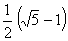Sol: Let geometric progression is a, ar, ar2,………….;(a, r > 0)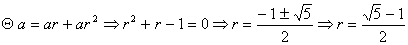Correct choice: (4) Q. 2. If sin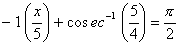then a value of x is. 1 3 4 5 Sol: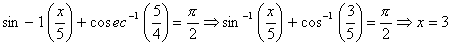Correct choice: (2) Q. 3. In the binomial expansion of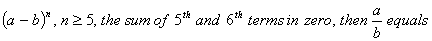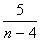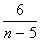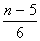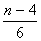Sol: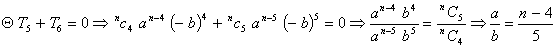orrect choice: (4)